# Test: Recurrence Relations

## 15 Questions MCQ Test Algorithms | Test: Recurrence Relations

Description
Attempt Test: Recurrence Relations | 15 questions in 35 minutes | Mock test for Computer Science Engineering (CSE) preparation | Free important questions MCQ to study Algorithms for Computer Science Engineering (CSE) Exam | Download free PDF with solutions
QUESTION: 1

### Consider the following recurrence relation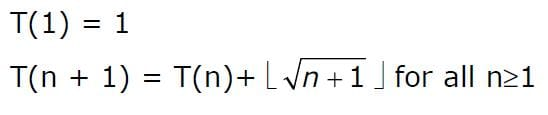The value of T(m2) for m ≥ 1 is

Solution:

One easy way to solve this is to try putting different
values of m.

For example, we know T(1) = 1. If we put m = 1, only A
and B satisfy the result.

m = 2

T(2) = T(1) + 1 = 2
T(3) = T(2) + 1 = 3
T(4) = T(3) + 2 = 5

Both A & B produce 5

m = 3
T(9) = T(4) + 2*5 + 1 = 5 + 10 + 1 = 16
Both A & B produce 16

m = 4
T(16) = T(9) + 3*7 + 1 = 16 + 21 + 1 = 38
Only B produces 38, A produces 34 which doesn't match

QUESTION: 2

### The solution to the recurrence equation T(2k) = 3 T(2k-1) + 1, T (1) = 1, is:

Solution:

We have T (2k) = 3  T (2k-1) + 1 = 32  T (2k-2) + 1 + 3 = 33  T (2k-3) + 1 + 3 + 9 . . . (k steps of recursion (recursion depth)) = 3k  T (2k-k) + (1 + 3 + 9 + 27 + ... + 3k-1) = 3k + ( ( 3k - 1 ) / 2 ) = ( (2 * 3k) + 3k - 1 )/2 = ( (3 * 3k) - 1 ) / 2 = (3k+1 - 1) / 2 Hence, B is the correct choice.   Please comment below if you find anything wrong in the above post.

QUESTION: 3

### Select the correct asymptotic complexity of an algorithm with runtime T(n, n) where T(x, c) = Θ(x) for c <= 2, T(c, y) = Θ(y) for c <= 2, and T(x, y) = Θ(x+y) + T(x/2, y/2)

Solution:

The recurrence is
T(x, y) = Θ(x+y) + T(x/2, y/2)
It can be written as below.
T(x, y) = Θ(x+y) + Θ((x+y)/2) + Θ((x+y)/4) +  Θ((x+y)/8)  .....
T(x, y) = Θ((x+y) + (x+y)/2 + (x+y)/4 + (x+y)/8  ..... )
The above expression forms a geometric series with ratio as 2 and starting element as (x+y)/2 T(x, y) is upper bounded by Θ(x+y) as sum of infinite series is 2(x+y). It is lower bounded by (x+y)

QUESTION: 4

Consider the recurrence relation a1 = 8, an = 6n2 + 2n + an-1. Let a99 = k x 104. The value of K is _____   Note : This question was asked as Numerical Answer Type.

Solution:

a1 = 8 an = 6n2 + 2n + an-1   an = 6[n2 + (n-1)2] + 2[n + (n-1)] + an-2   Continuing the same way till n=2, we get an = 6[n2 + (n-1)2 + (n-2)2 + ... + (2)2] + 2[n + (n-1) + (n-2) + ... + (2)] + a1   an = 6[n2 + (n-1)2 + (n-2)2 + ... + (2)2] + 2[n + (n-1) + (n-2) + ... + (2)] + 8   an = 6[n2 + (n-1)2 + (n-2)2 + ... + (2)2] + 2[n + (n-1) + (n-2) + ... + (2)] + 6 + 2   an = 6[n2 + (n-1)2 + (n-2)2 + ... + (2)2 + 1] + 2[n + (n-1) + (n-2) + ... + (2) + 1]   an = (n)*(n+1)*(2n+1) + (n)(n+1) = (n)*(n+1)*(2n+2)   an = 2*(n)*(n+1)*(n+1) = 2*(n)*(n+1)2   Now, put n=99. a99 = 2*(99)*(100)2 = 1980000 = K * 104 Therefore, K = 198.   Thus, C is the correct choice.

QUESTION: 5

When n = 22k for some k ≥ 0, the recurrence relation
T(n) = √(2) T(n/2) + √n, T(1) = 1
evaluates to :

Solution:

Please note that the question is asking about exact solution. Master theorem provides results in the form of asymptotic notations. So we can't apply Master theorem here. We can solve this recurrence using simple expansion or recurrence tree method.

T(n) = √(2) T(n/2) + √n = √(2) [√(2) T(n/4) + √(n/2) ] + √n = 2 T(n/4) + √2 √(n/2) +√n = 2[ √2 T(n/8) + √(n/4) ]+√2 √(n/2)+√n = √(2^3) T(n/8)+ 2 √(n/4) + √2 √(n/2) +√n = √(2^3) T(n/8)+√n +√n +√n = √(2^3) T(n/(2^3))+3√n ............................................. = √(2^k) T(n/(2^k))+k√n = √(2^logn) + logn √n = √n + logn √n = √n(logn +1)

Alternate Solution : This question can be easily done by substitution method look: T(1)= 1; GIVEN. Now use n=2 in the given recurrence relation which gives 2*(1.414) (since value of root over 2 is 1.414) now by looking at the options use n=2 which satisfies option A.

QUESTION: 6

Standard planning algorithms assume environment to be __________.

Solution:

Standard planning algorithms assume environment to be both deterministic and fully observable. So, option (A) is correct.

QUESTION: 7

The worst-case running time of Merge sort algorithm is described by the following recurrence relation :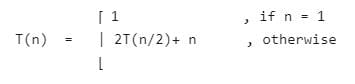Given recurrence equation evaluates to -

Solution:

T(n) = 2T (n/2) + n = 21 T(n/21) + 1.n [ k = 1] = 2k-1 T(n/2k-1) + (k-1)n = 2k-1 (2T(n/2k + n/2k-1) + (k-1)n = 2k T(n/2k) + kn

Option (A) is Correct.

QUESTION: 8

What is the time complexity for the following C module? Assume that n>0 . int module(int n) { if (n == 1) return 1; else return (n + module(n-1)); }

Solution:

F(n) = (n + f(n-1)) → n + (n-1 + f(n-2))→ n + (n-1 + (n-2 (+...+ (n-(n-1) + f(1))) Time complexity of of code is = O(n)

QUESTION: 9

For parameters a and b, both of which are ω(1), T(n)=T(n1/a)+1, and T(b)=1. Then T(n) is

Solution:

Given,

T(n) = T(n1/a)+1, T(b) = 1

Now, using iterative method,

= T(n) = [T(n1/a2)+1] + 1 = [T(n1/a3)+1] + 2 = [T(n1/a4)+1] + 3 . . . = [T(n1/ak)+1] + (k-1) = T(n1/ak) + k

Let,

→ n1/ak = b → log(n1/ak) = log(b) → ak = log(n) / log (b) = logb(n) → k = logalogb(n)

Therefore,

= T(n1/ak) + k = T(b) + logalogb(n) = 1 + logalogb(n) = Θ(logalogb(n))

Option (A) is correct.

QUESTION: 10

Consider the following three functions.

f1 = 10n

f2 = nlogn

f3 = n√n

Which one of the following options arranges the functions in the increasing order of asymptotic growth rate?

Solution:

On comparing power of these given functions : f1 has n in power. f2 has logn in power. f3 has √n in power. Hence, f2, f3, f1 is in increasing order. Note that you can take the log of each function then compare.

QUESTION: 11

Consider the following recurrence relation.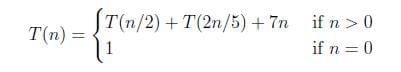Which one of the following options is correct?

Solution:

Given, recurrence relation can be written as, T(n) = T(n/2) + T(2n/5) + 7n T(n) = T((5/2)n/5) + T(2n/5) + 7n Since, sum of numerator (5/2+2 = 4.5) is less than denominator (5), so time complexity would be function itself. Hence, T(n) = Θ(7n) = Θ(n)

QUESTION: 12

What is the worst-case number of arithmetic operations performed by recursive binary search on a sorted array of size n?

Solution:

Arithmetic operations performed by binary search on sorted data items means computation of mid element required arithmetic operation. So it will be computed log(n) time and Hence option (C) will be correct.

QUESTION: 13

What is the value of following recurrence.

T(n) = T(n/4) + T(n/2) + cn2
T(1) = c
T(0) = 0
Where c is a positive constant

Solution:

Following is the initial recursion tree for the given recurrence relation.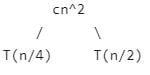If we further break down the expression T(n/4) and T(n/2), we get following recursion tree.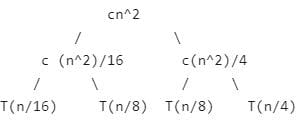Breaking down further gives us following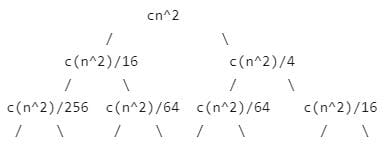To know the value of T(n), we need to calculate sum of tree nodes level by level. If we sum the above tree level by level, we get the following series T(n) = c(n^2 + 5(n^2)/16 + 25(n^2)/256) + ....

The above series is geometrical progression with ratio 5/16 To get an upper bound, we can sum the above series for infinite terms. We get the sum as (n^2) / (1 - 5/16) which is O(n^2)

QUESTION: 14

The running time of an algorithm is represented by the following recurrence relation:

if n <= 3 then T(n) = n

else T(n) = T(n/3) + cn

Which one of the following represents the time complexity of the algorithm?
(A)(n)
(B)(n log n)
(C)(n^2)
(D)(n^2log n)

Solution:

T(n) = cn + T(n/3)

= cn + cn/3 + T(n/9)

= cn + cn/3 + cn/9 + T(n/27)

Taking the sum of infinite GP series. The value of T(n) will be less than this sum.

T(n) = cn(1/(1-1/3))

= 3cn/2

or we can say

cn = T(n) = 3cn/2

Therefore T(n) =(n)

QUESTION: 15

Consider the following recurrence. T(n) = T(√n) + θ(Log Log n) What is the value of recurrence?
(A) θ( (Log Log n)2)
(B) θ(Log Log n)
(B) θ(n)
(B) θ(Log Log Log n)

Solution:

Change of variables: let m = lg n. Recurrence becomes S(m) = S(m/2) + θ(lgm). Case 2 of master’s theorem applies, so T(n) = θ( (log Log n) ^ 2).Use Code STAYHOME200 and get INR 200 additional OFF Use Coupon Code